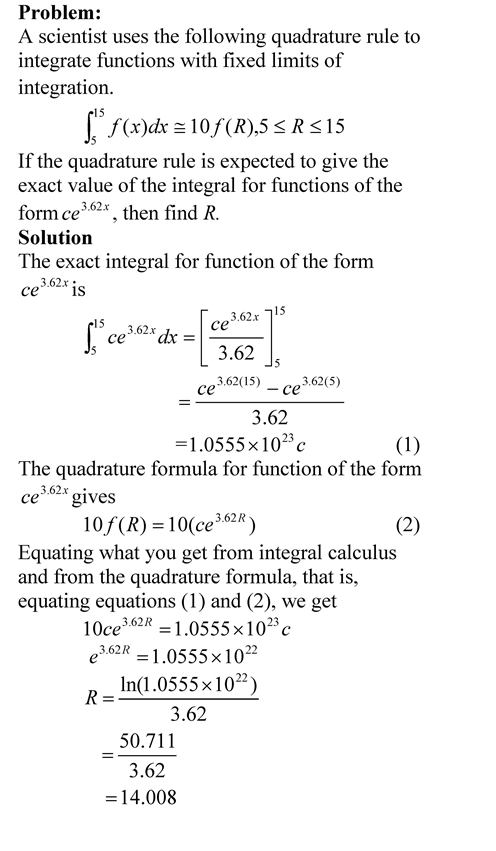To relate how quadrature formulas are derived, see a simple example of how to do so.

To relate how quadrature formulas are derived, see a simple example of how to do soSee the pdf file for solution.

______________________

This post is brought to you by

• Holistic Numerical Methods Open Course Ware:
• the textbooks on
• the Massive Open Online Course (MOOCs) available at

## Example to show that a polynomial of order n or less that passes through (n+1) data points is unique.

Problem: Through three data pairs (0,0), (3,9) and (4,12), an interpolating polynomial of order 2 or less is found to be y=3x. Prove that there is no other polynomial of order 2 or less that passes through these three points.

See the pdf file for solution.

______________________

This post is brought to you by

• Holistic Numerical Methods Open Course Ware:
• the textbooks on
• the Massive Open Online Course (MOOCs) available at